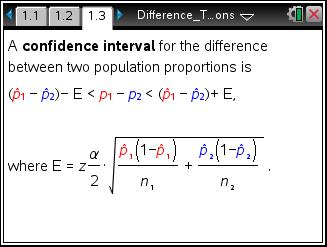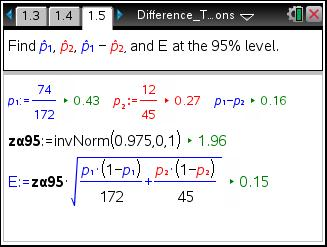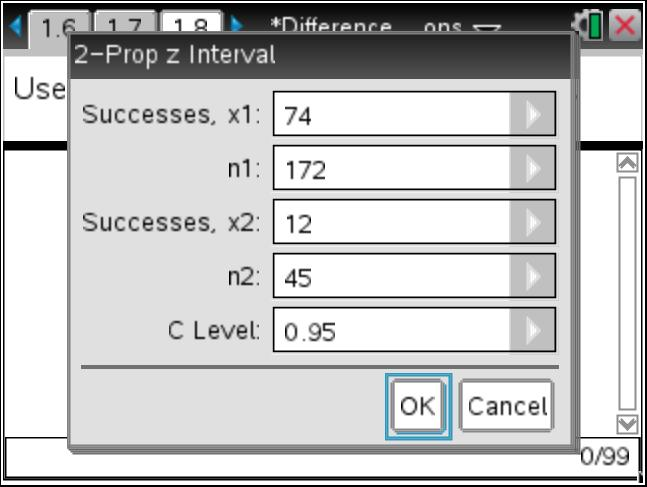# Activities

••• ##### Subject Area

• Math: Statistics: Inferential Statistics

• ##### Author9-12

40 Minutes

• ##### Device
•TI-Nspire™ CX/CX II
•TI-Nspire™ CX CAS/CX II CAS
• TI-Nspire™
• TI-Nspire™ CAS
• ##### Software

TI-Nspire™
TI-Nspire™ CAS

3.2

• ##### Other Materials
By Michele Patrick

## Difference Between Two Proportions

#### Activity Overview

Students use confidence intervals to estimate the difference of two population proportions. First they find the intervals by calculating the critical value and the margin of error. Then, they use the 2-propZInterval command. Students find confidence intervals for differences in proportions in real-life situations and use them to make judgments about certain claims. Last, they determine required sample size, n, when given a confidence interval and margin of error.

#### Key Steps

•To begin the activity, explain to students that they can estimate the true difference between two population proportions and that subscripts will be used to differentiate between the two populations. Then introduce the formulas for the margin of error and confidence interval.

•On page 1.7, students are to construct the confidence interval by subtracting from and adding to the difference of the sample proportions.

The screenshot shows the calculation of the interval two ways, one using the stored values and another entering the values directly.

•Let students check their work on page 1.10 by pressing MENU > Statistics > Confidence Intervals > 2-Prop z Interval and entering the required information.